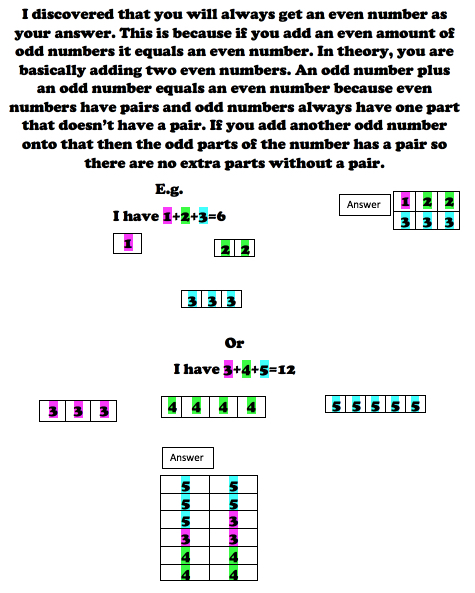#### You may also like### Consecutive Numbers

An investigation involving adding and subtracting sets of consecutive numbers. Lots to find out, lots to explore.### Roll These Dice

Roll two red dice and a green dice. Add the two numbers on the red dice and take away the number on the green. What are all the different possible answers?### Domino Square

Use the 'double-3 down' dominoes to make a square so that each side has eight dots.

# Take Three Numbers

##### Age 7 to 11Challenge Level

We had just a few submissions for this task but they all showed good understanding.

Maisie from  St Mary's C of E (Aided) Primary School sent in the following;

Two odds add one even always equal an even number but two evens add an odd will always equal an odd. Three evens will always equal an odd whereas three odds will always equal an odd too. I was wondering about this last sentence - what do you think?

Evelyn from Harrison Primary School sent in this good illustration of the whole idea;Susan from The Sacred Heart Primary School in Coventry wrote;

I know that even though I use 2 odd numbers and 1 even [3,7, 2 and 1,9,4] for both calculations they actually come up with different answers[12,14]. THE END!

Zuzanna from St Gregory's Catholic Primary School

Odd number add odd number add even number ALWAYS equals even number. For
example:
3 (odd) + 5 (odd) + 2 (even) = 10 (even).
7 + 9 + 10 = 26
1+3+2= 6

Thank you all for these contributions.